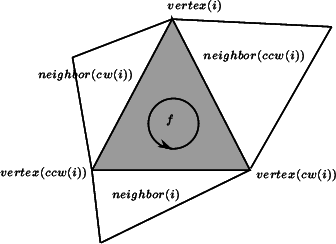CGAL 5.1 - 2D Triangulation
CGAL::Triangulation_cw_ccw_2 Class Reference

#include <CGAL/Triangulation_2.h>

Inherited by CGAL::Triangulation_2< Traits, Tds >.

## Definition

The class Triangulation_cw_ccw_2 offers two functions int cw(int i) and int ccw(int i) which, given the index of a vertex in a face, compute the index of the next vertex of the same face in clockwise or counterclockwise order.

This works also for neighbor indexes. Thus, for example the neighbor neighbor(cw(i)) of a face f is the neighbor which is next to neighbor(i) turning clockwise around f. The face neighbor(cw(i)) is also the first face encountered after f when turning clockwise around vertex i of f.

Many of the classes in the triangulation package inherit from Triangulation_cw_ccw_2. This is for instance the case for CGAL::Triangulation_2::Face. Thus, for example the neighbor neighbor(cw(i)) of a face f is the neighbor which is next to neighbor(i) turning clockwise around f. The face neighbor(cw(i)) is also the first face encountered after f when turning clockwise around vertex i of f.Vertices and neighbors.
CGAL::Triangulation_2
TriangulationDSFaceBase_2

## Creation

Triangulation_cw_ccw_2 ()
default constructor.

## Operations

int ccw (const int i) const
returns the index of the neighbor or vertex that is next to the neighbor or vertex with index i in counterclockwise order around a face.

int cw (const int i) const
returns the index of the neighbor or vertex that is next to the neighbor or vertex with index i in counterclockwise order around a face.